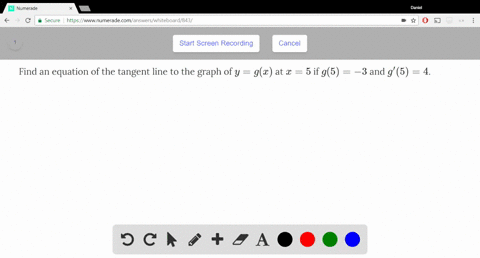Enroll in one of our FREE online STEM summer camps. Space is limited so join now!View Summer Courses### Find an equation of the tangent line to the graph…

01:41

Need more help? Fill out this quick form to get professional live tutoring.

Get live tutoring
Problem 19

For the function $f$ graphed in Exercise 18:

(a) Estimate the value of $f'(50)$.
(b) Is $f'(10) > f'(30)$?
(c) Is $f'(60) > \dfrac{f(80) - f(40)}{80 - 40}$? Explain.

a) $\approx 26$
b) The given statement is not true
c) The given statement is true

## Discussion

You must be signed in to discuss.

## Video Transcript

there's a problem. Number nineteen of the Stuart Calculus eighth edition, section two point seven for the function as craft in Exercise eighteen, which is here party estimate the value of F prime of fifty. The prime of primer fifty will be the ah derivative or the slope of the tangent line to the function F addicts Eagle fifty. We can estimate this using a second line very close to fifty. Let's say maybe from fifty to sixty. We see from fifty to sixty. That slope is approximately equal to the slip of the pen jawline. At fifteen, it increases three hundred over an increase of ex of ten on three hundred ten This thirty. So we're gonna ask to meet this value as approximately thirty part of me is at time of ten Created in the prime of thirty. Well, again after crime represents is the slope of the ah, the slope to the function the slope of the tangent line to the function at each of those points ten and dirty At ten. We have a negative so and that dirty we have a less thing. This it was not as steep so that as negative. So if we use numbers such as negative ten here for four f Pregnant in has an estimate. Then over here would be negative. Five perhaps. So we see clearly that because this is less steep in less negative, f prime of thirty must be greater than at prime of ten. So this is false. Poor part C is at prime of sixty Is a slipped a tendon line at sixty Greater than ah, the rate of change this difference and the function over the difference in the X values from forty eighty basically the average rate of change. We can look at this visually and look at the average rate of change here from forty eighty from forty to eighty, which is at the end. Here we have, ah line that goes this way. If you were to connect the line, that would be the second and the slope of that second is equal to this direction here. So it's a positive value. But if we were to imagine the slope of seek of the tangent line at X equal succeed, which is what this part is, this first term we can see it's a steeper it's much more positive. It is greater than the second, the slope of the secret here. So this is indeed true. And again because the slope of the tangent line had had sixty some of the tension line to the function, F at sixteen is much steeper than the average rate of change or of these soap, with seek in line from forty to eighty for the function f so this is true.